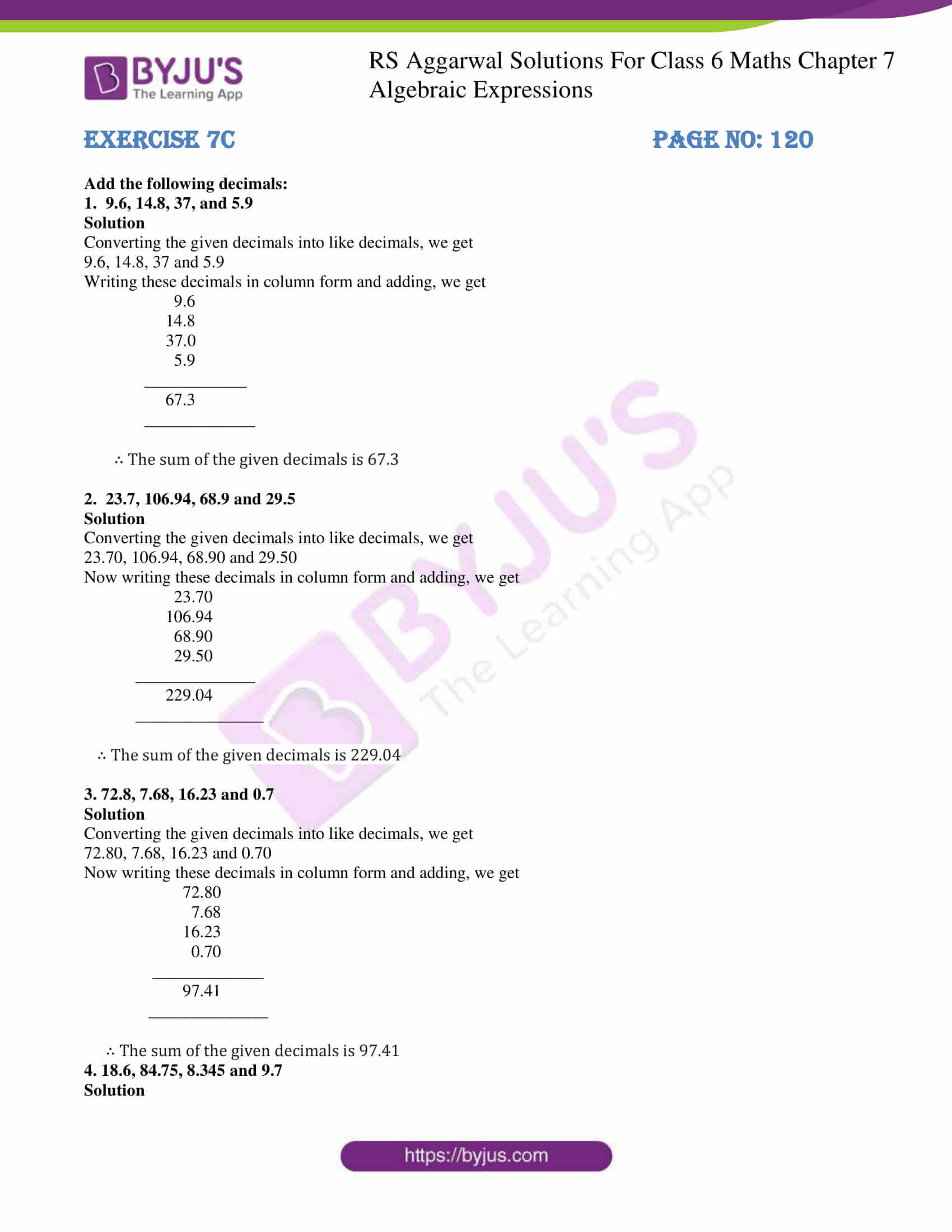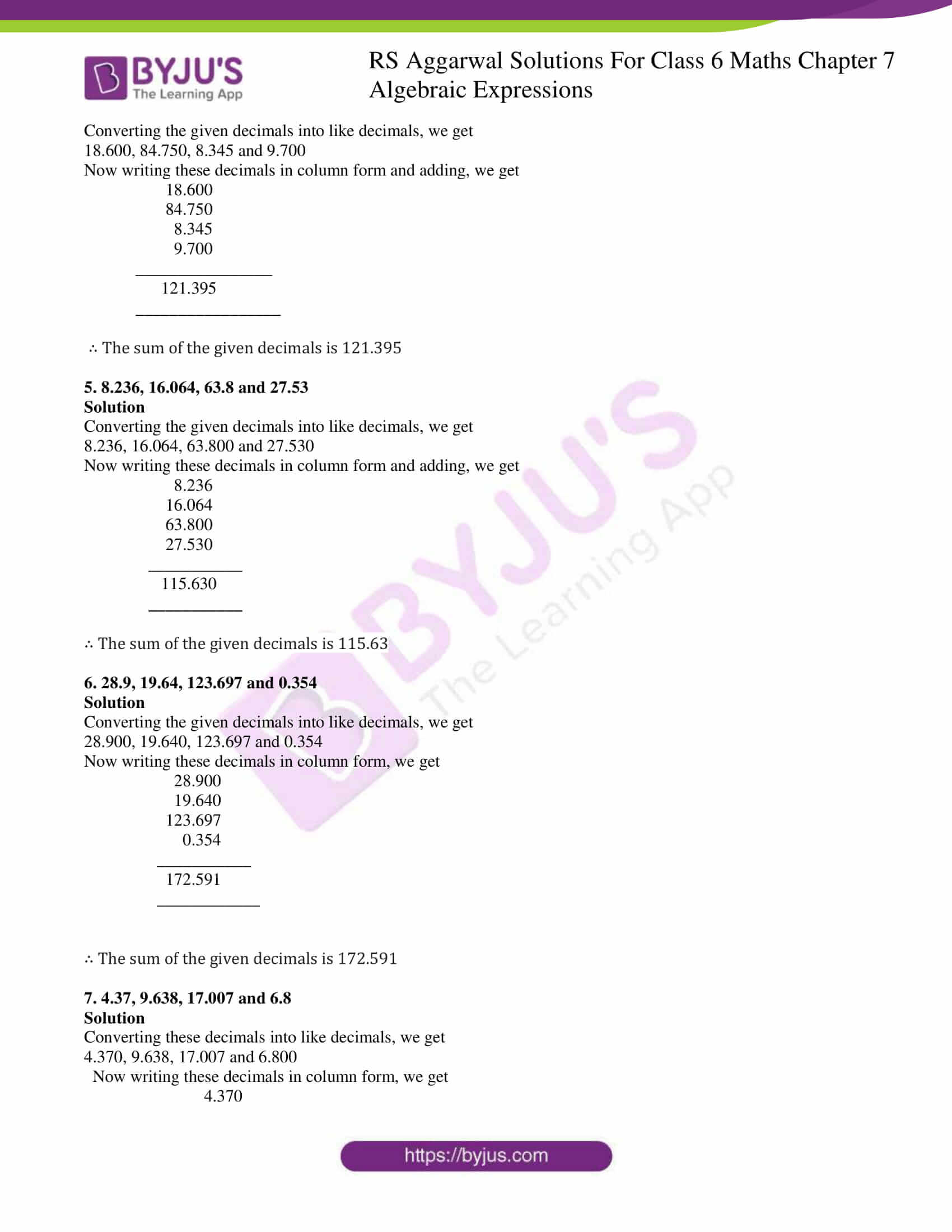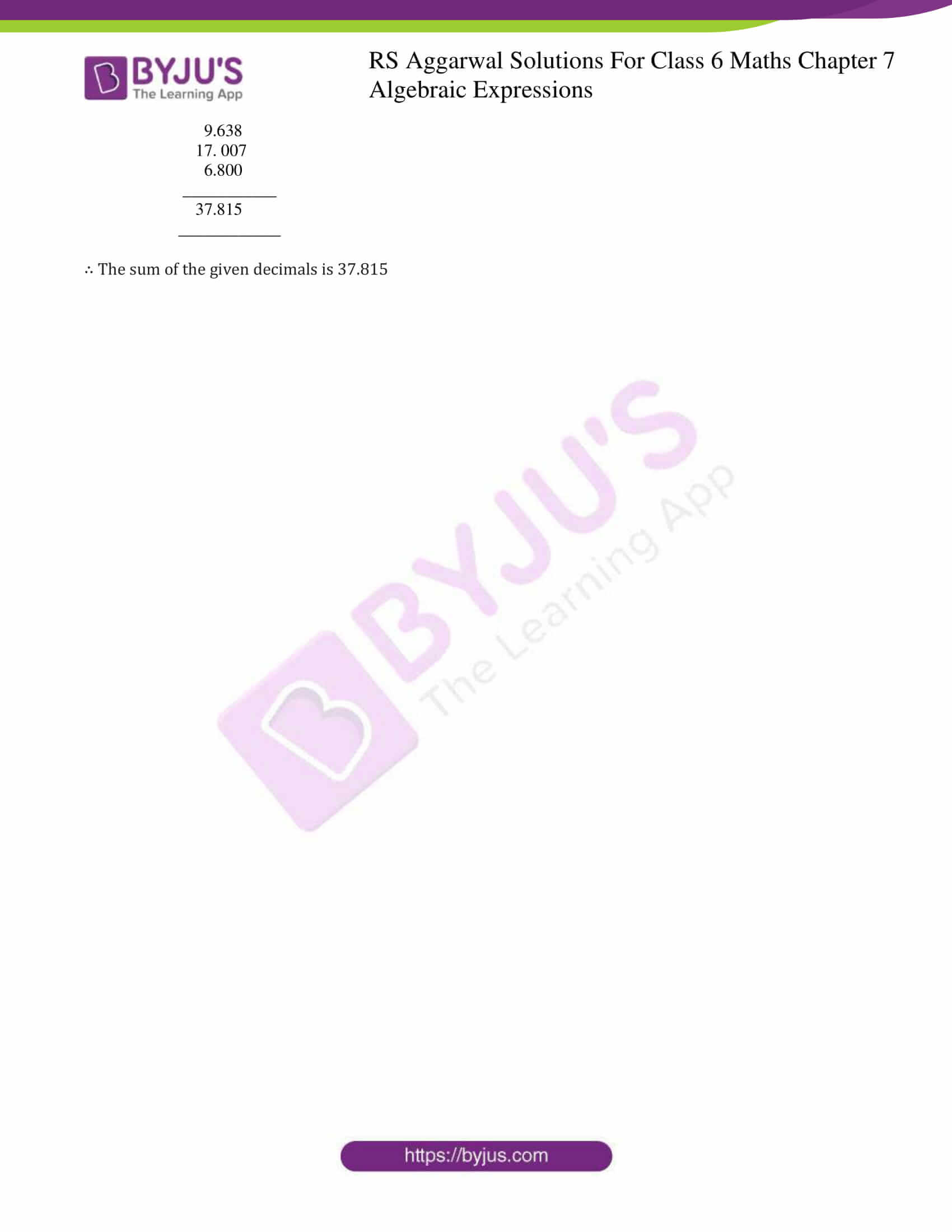# RS Aggarwal Solutions for Class 6 Chapter 7 Decimals Exercise 7C

RS Aggarwal Solutions for Class 6 Chapter 7 Exercise 7C are here in simple PDF format for free download. Mathematics experts of BYJU’S design RS Aggarwal Solutions with accurate answers. The students can practice exercise wise problems of RS Aggarwal textbook to perform well in the exam. Exercise 7C provides knowledge about addition operation on Decimals. Students can gain better knowledge about the topic by going through RS Aggarwal Solutions for Class 6.

## Download PDF of RS Aggarwal Solutions for Class 6 Chapter 7 Decimals Exercise 7C### Access answers to Maths RS Aggarwal Solutions for Class 6 Chapter 7 Decimals Exercise 7C

1. 9.6, 14.8, 37, and 5.9

Solution

Converting the given decimals into like decimals, we get

9.6, 14.8, 37 and 5.9

Writing these decimals in column form and adding, we get

9.6

14.8

37.0

5.9

____________

67.3

_____________

∴ The sum of the given decimals is 67.3

2. 23.7, 106.94, 68.9 and 29.5

Solution

Converting the given decimals into like decimals, we get

23.70, 106.94, 68.90 and 29.50

Now writing these decimals in column form and adding, we get

23.70

106.94

68.90

29.50

______________

229.04

_______________

∴ The sum of the given decimals is 229.04

3. 72.8, 7.68, 16.23 and 0.7

Solution

Converting the given decimals into like decimals, we get

72.80, 7.68, 16.23 and 0.70

Now writing these decimals in column form and adding, we get

72.80

7.68

16.23

0.70

_____________

97.41

______________

∴ The sum of the given decimals is 97.41

4. 18.6, 84.75, 8.345 and 9.7

Solution

Converting the given decimals into like decimals, we get

18.600, 84.750, 8.345 and 9.700

Now writing these decimals in column form and adding, we get

18.600

84.750

8.345

9.700

________________

121.395

_________________

∴ The sum of the given decimals is 121.395

5. 8.236, 16.064, 63.8 and 27.53

Solution

Converting the given decimals into like decimals, we get

8.236, 16.064, 63.800 and 27.530

Now writing these decimals in column form and adding, we get

8.236

16.064

63.800

27.530

___________

115.630

___________

∴ The sum of the given decimals is 115.63

6. 28.9, 19.64, 123.697 and 0.354

Solution

Converting the given decimals into like decimals, we get

28.900, 19.640, 123.697 and 0.354

Now writing these decimals in column form, we get

28.900

19.640

123.697

0.354

___________

172.591

____________

∴ The sum of the given decimals is 172.591

7. 4.37, 9.638, 17.007 and 6.8

Solution

Converting these decimals into like decimals, we get

4.370, 9.638, 17.007 and 6.800

Now writing these decimals in column form, we get

4.370

9.638

17. 007

6.800

___________

37.815

____________

∴ The sum of the given decimals is 37.815